>  Test: BITSAT Mock Test- 11

# Test: BITSAT Mock Test- 11

Test Description

## 150 Questions MCQ Test | Test: BITSAT Mock Test- 11

 1 Crore+ students have signed up on EduRev. Have you?
Test: BITSAT Mock Test- 11 - Question 1

### When a radioactive substance emits an α-particle, its position in the Periodic Table is lowered by

Test: BITSAT Mock Test- 11 - Question 2

### In a transformer, the number of turns in primary coil and secondary coil are 5 and 4 respectively. If 240 V is applied on the primary coil, then the ratio of current in primary and secondary coil is

Test: BITSAT Mock Test- 11 - Question 3

### A battery of e.m.f. 6 V and internal resistance 4 Ω is connected to a external resistor. If the current in the circuit is 0.5 A, then terminal voltage of battery when the circuit is closed, is

Test: BITSAT Mock Test- 11 - Question 4
Which of the following spectral series in hydrogen atom give spectral line of 4860 Å
Test: BITSAT Mock Test- 11 - Question 5
When we touch the terminals of a high voltage capacitor, even after a high voltage has been cut off, then the capacitor has a tendency to
Test: BITSAT Mock Test- 11 - Question 6
A charge q is located at the centre of a cube. The electric flux through any face is
Test: BITSAT Mock Test- 11 - Question 7
Which of the following statement is not correct
Test: BITSAT Mock Test- 11 - Question 8
The resistance of each arm of the Wheatstone's bridge is 10 ohm. A resistance of 10 ohm is connected is series with galvanometer then the equivalent resistance across the battery will be
Test: BITSAT Mock Test- 11 - Question 9
The number of turs of primary and secondary coils of a transformer are 5 and 10 respectively and mutual inductance of the transformer is 25 H. Now, number of turns in primary and secondary are made 10 and 5 respectuvely. Mutual inductance of transformer will be
Test: BITSAT Mock Test- 11 - Question 10

A horizontal force, just sufficient to move a body of mass 4kg lying on a rough horizontal surface is applied on it. The coefficients of static and kinetic friction between the body and the surface are 0.8 and 0.6 respectively. If the force continues to act even after the block has started moving, the acceleration of the block in ms−2 is [g = 10 m s − 2 ]

Test: BITSAT Mock Test- 11 - Question 11

What will be the ratio of de Broglie wavelengths of proton and α-particle of same energy

Detailed Solution for Test: BITSAT Mock Test- 11 - Question 11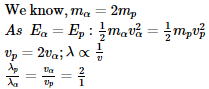Test: BITSAT Mock Test- 11 - Question 12
Which of the following statements is true
Test: BITSAT Mock Test- 11 - Question 13
Two thermometers A and B are exposed in sun light. The valve of A is painted black, But that of B is not painted. The correct statement regarding this case is
Test: BITSAT Mock Test- 11 - Question 14

The pressure and temperture of an ideal gas in a closed vessel are 720 kPa and 40oC respectively. If 1/4th of the gas is released from the vessel and the temperature of the remaining gas is raised to 353oC, the final pressure of the gas is

Test: BITSAT Mock Test- 11 - Question 15
Two wires of same length are shaped into a square and a circle. If they carry same current, ratio of the magnetic moments is
Test: BITSAT Mock Test- 11 - Question 16
A bar magnet of magnetic moment M and length L is cut into two equal parts each of length L/2. The magnetic moment of each part will be
Test: BITSAT Mock Test- 11 - Question 17

A spherical drop of water has 1 mm radius. If the surface tension of water is 70 x 10⁻3 N-m⁻1, then difference of pressures between inside and outside of spherical drop is

Test: BITSAT Mock Test- 11 - Question 18

How much force is required to produce an elongation of 0.2% in the length of a brass wire of diameter 0.6 mm? (Take Young's modulus for brass = 0.9 x 1011 N-m⁻2)

Test: BITSAT Mock Test- 11 - Question 19
Water rises to a height of 5 mm in a glass capillary tube. If the area of cross-section of the tube is reduced to one-sixteenth of the former value, then water rises to a height of
Test: BITSAT Mock Test- 11 - Question 20

A body falls from rest in the gravitational field of the earth. The distance travelled in the fifth second of its motion is (g=10 m/s2)

Test: BITSAT Mock Test- 11 - Question 21
A particle is kept at rest at the top of a sphere of diameter 42 m. When disturbed slightly, it slides down. At what height 'h' from the bottom, the particle will leave the sphere
Test: BITSAT Mock Test- 11 - Question 22
A 100 kg car is moving with a maximum velocity of 9 m/s across a circular track of radius 30 m. The maximum force of friction between the road and the car is
Test: BITSAT Mock Test- 11 - Question 23

A boy having a mass equal to 40 kilograms is standing in an elevator. The force felt by the feet of the boy will be greatest when the elevator (g=9.8 metres/sec2)

Test: BITSAT Mock Test- 11 - Question 24
The ability of eye to focus near as well as distant objects is called
Test: BITSAT Mock Test- 11 - Question 25
One of the two clocks on the earth is controlled by a pendulum and other by a spring. If both the clocks are taken to the moon, then which clock will have same time period as that on the earth?
Test: BITSAT Mock Test- 11 - Question 26

If a spring extends by x on loading then energy stored in the spring is (if T is the tension and k is the force constant of the spring)

Test: BITSAT Mock Test- 11 - Question 27

When a mass is rotating in a plane about a fixed point, its angular momentum is directed along

Test: BITSAT Mock Test- 11 - Question 28
A convex lens of focal length 0.5 m and concave lens of focal length 1 m are combined .The power of resulting lens will be
Test: BITSAT Mock Test- 11 - Question 29
If a solid spherical ball is rolling on a horizontal table, the ratio of its rotational kinetic energy to the total energy is
Test: BITSAT Mock Test- 11 - Question 30
A solid sphere is rotating in a free space. If radius of the sphere is increased, keeping the mass same, which one of the following will not be affected?
Test: BITSAT Mock Test- 11 - Question 31
A heater coil is cut into two equal parts and only one part is now used in the heater. Now the heat generated will become
Test: BITSAT Mock Test- 11 - Question 32
A perfect gas contained in a cylinder is kept in vacuum. If the cylinder suddenly bursts, then the temperature of the gas
Test: BITSAT Mock Test- 11 - Question 33

Dimensions of kinetic energy are

Test: BITSAT Mock Test- 11 - Question 34
Two samples A and B of a gas initially at the same pressure and temperature are compressed from volume V to V/2 (A isothermally and B adiabatically). The final pressure of A is
Test: BITSAT Mock Test- 11 - Question 35
The golden view of sea shell is due to
Test: BITSAT Mock Test- 11 - Question 36
Bubble's of colourless soap solution appear coloured in sun light. This is due to
Test: BITSAT Mock Test- 11 - Question 37
Two waves of same frequency and intensity superimpose with each other in opposite phases, then after superposition the
Test: BITSAT Mock Test- 11 - Question 38
16 tuning forks are arranged in increasing order of frequency. Any two consecutive tuning forks when sounded together produce 8 beats per second. If the frequency of the last tuning fork is twice that of the first, the frequency of the first tuning fork is
Test: BITSAT Mock Test- 11 - Question 39
An engine pumps up 100 kg of water through a height of 10 m in 5s. Given that the efficiency of engine is 60%. If g = 10 ms-2, the power of the engine is
Test: BITSAT Mock Test- 11 - Question 40
A 1.0 HP motor pumps out water from a well of depth 20m and fills a water tank of volume 2238 litres at a height of 10m from the ground. The running time of the motor to fill the empty water tank is
Test: BITSAT Mock Test- 11 - Question 41
The reaction of Ethanol with H₂SO₄ does not give
Test: BITSAT Mock Test- 11 - Question 42
Catalyst used in Rosenmund reduction is
Test: BITSAT Mock Test- 11 - Question 43
Bohr's model was modified by
Test: BITSAT Mock Test- 11 - Question 44

The angular momentum of an electron present in the excited state of hydrogen is 1.5h/ π . The electron is present in

Test: BITSAT Mock Test- 11 - Question 45

The number of atoms contained in 11.2 L of SO₂ at S.T.P. are

Test: BITSAT Mock Test- 11 - Question 46
Among the following pairs, the one which illustrates the law of multiple proportions is
Test: BITSAT Mock Test- 11 - Question 47
In the process of nitration, the electrophile formed is
Test: BITSAT Mock Test- 11 - Question 48
The reaction of aniline with benzaldehyde is
Test: BITSAT Mock Test- 11 - Question 49
The substances which act as emulsifiers in lipid metabolism are
Test: BITSAT Mock Test- 11 - Question 50
The vitamin which is formed in the skin by ultra-violet light is
Test: BITSAT Mock Test- 11 - Question 51
The compound which reacts with Fehling solution is
Test: BITSAT Mock Test- 11 - Question 52
Pick up the molecule with sp3 hybridised atom
Test: BITSAT Mock Test- 11 - Question 53

(ΔH - ΔU) for formation of carbon monoxide (CO) from its elements at 298 K is (R = 8.314 KJ-1 mol-1)

Detailed Solution for Test: BITSAT Mock Test- 11 - Question 53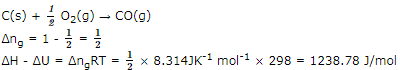Test: BITSAT Mock Test- 11 - Question 54
Which of the following favours the backward reaction in a chemical equilibrium?
Test: BITSAT Mock Test- 11 - Question 55
The rate constant is given by the equation K = P Ze(-E/RT). Which factor should register a decrease for the reaction to proceed more rapidly?
Test: BITSAT Mock Test- 11 - Question 56
Plants and living beings are the examples of
Test: BITSAT Mock Test- 11 - Question 57

A compound contains atoms of three elements A, B and C. If the oxidation number of A=+2, B=+5 and C= -2, the possible formula of the compound is

Test: BITSAT Mock Test- 11 - Question 58
The drug that is antipyretic as well as analgesic, is
Test: BITSAT Mock Test- 11 - Question 59
The colour of CoCl₃.5NH₃.H₂O is
Test: BITSAT Mock Test- 11 - Question 60
Which of the following transition metal shows only +3 oxidation state?
Detailed Solution for Test: BITSAT Mock Test- 11 - Question 60 La, Gd and Lu exhibit +3 oxidation state only because by loosing three electrons, they acquire stable configurations of empty, half-filled and completely filled 4f-subshells.
Test: BITSAT Mock Test- 11 - Question 61

The aqueous solution containing which of the following ions will be colourless?

Test: BITSAT Mock Test- 11 - Question 62

Oxidation number of S in H₂S₂O₈ is

Test: BITSAT Mock Test- 11 - Question 63

Among the following compounds (I-III), the correct order in reaction with electrophile is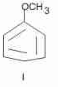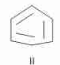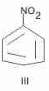Test: BITSAT Mock Test- 11 - Question 64

Benzene on treatment with a mixture of conc.HNO₃ and conc.H₂SO₄ at 100oC gives

Test: BITSAT Mock Test- 11 - Question 65
The hair dyes available in the market generally contain two bottles,one containing dye and the other hydrogen peroxide .Before applying the dye , the two solutions are mixed. The hydrogen peroxide
Test: BITSAT Mock Test- 11 - Question 66

The ionic product of water will increase if

Detailed Solution for Test: BITSAT Mock Test- 11 - Question 66

The product of the concentration of hydronium ions and hydroxyl ion in pure water or in any acidic or basic solution is constant at a particular temperature.
It is known as ionic product of water.
It is designated as Kw
The value of Kw increases with the increase in temperature

Test: BITSAT Mock Test- 11 - Question 67
The number of possible structural isomers of C₄H₁₀O is
Test: BITSAT Mock Test- 11 - Question 68
Which of the following compounds is used as a refrigerant?
Test: BITSAT Mock Test- 11 - Question 69
Which of the following is obtained when N₂ reacts with calcium carbide?
Test: BITSAT Mock Test- 11 - Question 70
Which of the following can be used as anaesthesia?
Test: BITSAT Mock Test- 11 - Question 71
Higher percentage of carbon is found in
Test: BITSAT Mock Test- 11 - Question 72
On heating KClO₃, we get
Test: BITSAT Mock Test- 11 - Question 73
When potassium ferrocyanide crystals are heated with conc. H₂SO₄, the gas evolved is
Test: BITSAT Mock Test- 11 - Question 74
Which of the following is called a polyamide?
Test: BITSAT Mock Test- 11 - Question 75
What are the products formed when Li2CO3 undergoes decomposition?
Test: BITSAT Mock Test- 11 - Question 76
Which of the following is not correct?
Test: BITSAT Mock Test- 11 - Question 77
5 ml of N HCl, 20 ml of N/2 H₂SO₄ and 30 ml N/3 HNO₃ are mixed together, the volume made to one litre. The normality of the resulting solution is
Test: BITSAT Mock Test- 11 - Question 78

If two moles of an ideal gas at 546 K occupy volume of 44.8 L, then pressure must be

Test: BITSAT Mock Test- 11 - Question 79
7 grams of CO on complete oxidation produce the following weight of CO2
Test: BITSAT Mock Test- 11 - Question 80
Which is used for ending charge on colloidal solution of arsenic sulphide sol?
Test: BITSAT Mock Test- 11 - Question 81
The area between the curve $y=1-|x|$ and x-axis is
Test: BITSAT Mock Test- 11 - Question 82

If |x|<1, then the coefficient of xn in expansion of (1+x+x2+x3+...)2 is

Test: BITSAT Mock Test- 11 - Question 83
If two circles of equal radii a and with centres (2,3) and (5,6) cut each other at right angle then, a=
Test: BITSAT Mock Test- 11 - Question 84

If the line 2x - y + k = 0 is a diameter of the circle x2 + y2 + 6x -6y + 5 =0, then k is equal to

Test: BITSAT Mock Test- 11 - Question 85
Let z,w be complex numbers such that z̅ + iw ̅ = 0 and arg zw = π.Then arg z equals
Test: BITSAT Mock Test- 11 - Question 86
Solution of (x+y-1) dx+(2x+2y-3) dy = 0 is
Test: BITSAT Mock Test- 11 - Question 87

The degree of the differential equation d2y/dx2+(dy/dx)2+6y=0 is

Test: BITSAT Mock Test- 11 - Question 88

The solution of the equation (cosecx logy)dy+(x2y)dx=0 is

Test: BITSAT Mock Test- 11 - Question 89

If f(x)=cosx, 0≤x≤(π/2), the value of 'c' for which f(x) satisfies Mean Value theorem is

Test: BITSAT Mock Test- 11 - Question 90

For real values of x the minimum value of [(1-x+x2)/(1+x+x2)] is

Test: BITSAT Mock Test- 11 - Question 91
5/(1.2.3) + 7/(3.4.5) + 9/(5.6.7) + ..... is equal to
Test: BITSAT Mock Test- 11 - Question 92

The area enclosed between the curve y2 = 4x and the line y = x is

Test: BITSAT Mock Test- 11 - Question 93

If f g (x) dx = g (x), then f g (x) (f(x) + f'(x)) dx is equal to

Test: BITSAT Mock Test- 11 - Question 94
A flag staff on the top of a house subtends the same angle α at two points distance a and b from the house on the same side of it. Then the length of flag-staff is
Test: BITSAT Mock Test- 11 - Question 95

The number of normals to the hyperbola x2 ∕ a2 − y2 ∕ b2 = 1 from an external point is

Test: BITSAT Mock Test- 11 - Question 96

If cos⁻1(3/5)-sin⁻1(4/5)=cos⁻1x, then x=

Test: BITSAT Mock Test- 11 - Question 97
The set of all points, where the function
f(x) = [x/(1 + ∣x∣)] is differentiable, is
Test: BITSAT Mock Test- 11 - Question 98

If A and B are square matrices and A⁻1 and B⁻1 of same order exists, then (AB)⁻1is equal to

Test: BITSAT Mock Test- 11 - Question 99

If A and B are two matrices such that AB = B and BA = A, then A2 + B2 =

Test: BITSAT Mock Test- 11 - Question 100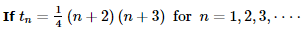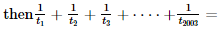Test: BITSAT Mock Test- 11 - Question 101

The maximum value of xy subject to x + y = 7 is

Test: BITSAT Mock Test- 11 - Question 102

Equation y2-x2+2x-1=0 represents

Test: BITSAT Mock Test- 11 - Question 103

If ω is an real cube root of unity, then (a+b)(a+bω)(a+bω2) is

Test: BITSAT Mock Test- 11 - Question 104

Two tangents are drawn from the point (-2,-1) to the parabola y2=4x. If α is the angle between them, then tan α =

Test: BITSAT Mock Test- 11 - Question 105

The angle between the tangents drawn from the origin to the parabola y2=4z(x-a) is

Test: BITSAT Mock Test- 11 - Question 106

There are 16 players in a cricket team in which 5 are ballers and 2 are wicket keepers. How many ways of 11 players can be made from them which have 3 ballers and 1 wicket keeper?

Detailed Solution for Test: BITSAT Mock Test- 11 - Question 106

We are to choose 11 players, including at least 3 bowlers and 1 wicket keeper.
out of 16 players, number of bowlers = 5 and the number of wicket keepers = 2
others = 16 - (5 + 2) = 9
now the required selection can be done in following manner:
3B + 1W + 7Ot (i) case or 3B + 2W + 6Ot (ii) case or 4B + 1W + 6Ot (iii) case or 4B + 2W + 5Ot (iv) case
or 5B + 1W + 5Ot (v) case or 5B + 2W + 4Ot (vi) case
= 5C3 . 2C1 . 9C7 + 5C3 . 2C2 . 9C6 + 5C4 . 2C1 . 9C6 + 5C4 . 2C2 . 9C5 + 5C5 . 2C1 . 9C5 + 5C5 . 2C2 . 9C4
= 10 * 2 * 36 + 10 * 1 * 84 + 5 * 2 * 84 + 5 * 1 * 126 + 1 * 2 * 126 + 1 * 1 * 126
= 720 + 840 + 840 + 1008 = 3408

Test: BITSAT Mock Test- 11 - Question 107
There are 10 cages for keeping 10 animals in a circus in which 10 cages are so small that 5 animals out of 10 can not enter in to them. In how many ways can 10 animals be kept in 10 cages?
Test: BITSAT Mock Test- 11 - Question 108
A rifleman is firing at a distance target and has only 10% chance of hitting it. The number of rounds, he must fire in order to have more than 50% chance of hitting it, at least once is
Detailed Solution for Test: BITSAT Mock Test- 11 - Question 108
Let n be the number of rounds, then Required Probability, is1/10 + 9/10 x 1/10 + (9/10)^2 x 1/10 + (9/10)^3 x 1/10 +... + (9^10)^n x 1/10 > 50%1/10 + 9/10 x 1/10 + (9/10)^2 x 1/10 + (9/10)^3 x 1/10 + ...+ (9/10)^n x 1/10 > 12
Its a geometric series of n terms, and we have to solve the inequality using the sum of n terms of a GP.(9/10)^n > 12⇒ n = 7
Probability would be more than 50% if atleast 7 rounds are fired.
Test: BITSAT Mock Test- 11 - Question 109

In Δ A B C , r + r3 + r1 − r2 =

Test: BITSAT Mock Test- 11 - Question 110

A committee of five is to be chosen from a group of 9 people. The probability that a certain married couple will either serve together or not at all is

Detailed Solution for Test: BITSAT Mock Test- 11 - Question 110

The total number of ways in which 5 person can be chosen out of 9 person is 9C5 = 126
The couple serves the committee in 7C3 * 2C2 = 35 ways
The couple does not serve the committee in
7C5 - 7C2 = 21 ways
Since the couple will either be together or not at all
∴ favourable number of cases = 35 + 21 = 56
∴ required porbability = 56/126 = 4/9

Test: BITSAT Mock Test- 11 - Question 111

In Δ A B C , if the line joining the circumcentre 'O' and the incentre I is parallel to BC ,then cosB + cosC =

Test: BITSAT Mock Test- 11 - Question 112

The quadratic equation whose roots are three times the roots of the equation 3 ax2 + 3 bx + c = 0 is

Test: BITSAT Mock Test- 11 - Question 113
If a, b, c are in H.P., then straight line x/a + x/b + 1/c = 0 always passes thro' a fixed point and that point is
Test: BITSAT Mock Test- 11 - Question 114
If A and B are two sets such that n(A) = 70, n(B) = 60 and n(A ∪ B)= 110, then n (A ∩ B) is equal to
Test: BITSAT Mock Test- 11 - Question 115
If aN = {ax : x ∈ N} and bN ∩ cN = dN, where b, c ∈ N are relatively prime, then
Test: BITSAT Mock Test- 11 - Question 116

if the 10th term of a G.P.is 9 and 4th term is 4, then its 7th term is

Detailed Solution for Test: BITSAT Mock Test- 11 - Question 116

The terms of a G.P. are of form a, ar, ar2, ar3 ,.... ar9 = 9
So, r9 = 9 a .....(1)
ar3 = 4 .....(2)
Cubing both sides of (2), we get,
a3r9 = 64
putting value of r9 in this, we get,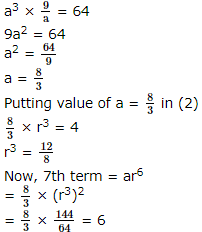Test: BITSAT Mock Test- 11 - Question 117
The area of triangles formed by lines x=0, y=0 and (x/a) + (y/b) = 1 is
Test: BITSAT Mock Test- 11 - Question 118
The subnormal to the curve xy = c2 at any point varies directly as
Test: BITSAT Mock Test- 11 - Question 119
The distance of the point (3,4,5) from y-axis is
Test: BITSAT Mock Test- 11 - Question 120
If sinθ=√3cosθ, -π<θ<0, θ=
Test: BITSAT Mock Test- 11 - Question 121
The plane x - 2y + z - 6 = 0 and the line (x/1) = (y/2) = (z/3) are related as the line
Test: BITSAT Mock Test- 11 - Question 122
If tan (cotx) = cot(tanx), then sin2x is equal to
Test: BITSAT Mock Test- 11 - Question 123

[(2 sinθ tanθ(1-tanθ)+2 sinθ sec2θ)/(1+tanθ)2]=

Test: BITSAT Mock Test- 11 - Question 124

Let a=(1,-1,1),c=(-1,-1,0) be two vectors, then the vector b which satisfies a*b=c and a.b=1 is

Test: BITSAT Mock Test- 11 - Question 125

If a,b,c are three non-coplanar vectors, then [a+b,b+c,c+a]=-

Test: BITSAT Mock Test- 11 - Question 126
Find the Antonym of the word occurring in the sentence in capital letter as per the context.
Because of the failure of the monsoon, there was PAUCITY of foodgrains.
Detailed Solution for Test: BITSAT Mock Test- 11 - Question 126 Meaning of Paucity
Smallness of number; fewness
Scarcity; dearth
Synonyms : Scarcity, lack, shortage, rarity, insufficiency
Antonyms : abundance, affluence, plenty
Test: BITSAT Mock Test- 11 - Question 127
Fill in the blank with appropriate word.
The old 'Nature Versus.......' debate regarding crime continues even today.
Test: BITSAT Mock Test- 11 - Question 128
Find the antonym of OBLIQUITY.
Test: BITSAT Mock Test- 11 - Question 129
Choose the one which can be substituted for the given words/sentences
Hater of learning and knowledge.
Test: BITSAT Mock Test- 11 - Question 130
Fill in the blank with appropriate word.
Contemporary economic development differs.....from the Industrial Revolution of the 19th century.
Test: BITSAT Mock Test- 11 - Question 131
Choose the one which can be substituted for the given words/sentences
Person who believes that God is every thing and everything is God
Test: BITSAT Mock Test- 11 - Question 132
Spot the error.
Test: BITSAT Mock Test- 11 - Question 133
Spot the error.
Test: BITSAT Mock Test- 11 - Question 134
Find the Synonym of the word occurring in the sentence in capital letter as per the context.
People fear him because of his VINDICTIVE nature.
Detailed Solution for Test: BITSAT Mock Test- 11 - Question 134 'Vindictive' means revengeful
Test: BITSAT Mock Test- 11 - Question 135
Find the Synonym of the word occurring in the sentence in capital letter as per the context.
I can no longer be satisfied with FICTITIOUS good conduct.
Detailed Solution for Test: BITSAT Mock Test- 11 - Question 135 Meaning of FICTITIOUS - not genuine or authentic; assumed; false to give a fictitious address of, related to, or characteristic of fiction; created by the imagination
Test: BITSAT Mock Test- 11 - Question 136
Choose the word which is least like the other words in the group .
Test: BITSAT Mock Test- 11 - Question 137
Spot the error.
Test: BITSAT Mock Test- 11 - Question 138
Complete the analogous pair.
Carpet : Floor :: ? : Wall
Test: BITSAT Mock Test- 11 - Question 139

Group the following figures into three classes on the basis of identical properties.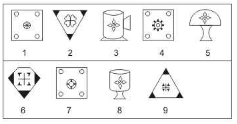Detailed Solution for Test: BITSAT Mock Test- 11 - Question 139

3,5,8 have similar designs (four leaves placed close to a small circle and forming a symmetrical design at the centre of the figure).
2,6,9 have similar designs (three of the corner of the main figure are shaded blace and there is a pattern formed around a '+' sign at the centre of the figure).
1,4,7 have similar designs (there are four small circles at the corners of the main figure and there is a wheal shaped element at the centre of the figure).

Test: BITSAT Mock Test- 11 - Question 140
Find out the term in the number series which is wrong.
89, 78, 86, 80, 85, 82, 83
Test: BITSAT Mock Test- 11 - Question 141

Find the missing character.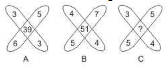Detailed Solution for Test: BITSAT Mock Test- 11 - Question 141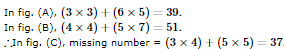Test: BITSAT Mock Test- 11 - Question 142
Find out the term in the number series which is wrong.
2, 3, 5, 8, 13, 20, 34
Test: BITSAT Mock Test- 11 - Question 143

'As a farmer , I was determined that we should get on the computer bandwagon ', says Krishnappa Jagannath, the 40-year old head of the Gram Panchayat who initiated the whole process three years ago. With tips from a computer-savvy brother in the US, Jagannath drew up a plan and convinced others of its merit. Within no time, he was able to raise Rs.65,000 from the public, enough for the purchase of two assembled Pentium II computers . That was easy enough. But who would operate these machines ? Jagannath decided that it had to be the villagers themselves.He got in touch with a software company , which agreed to train the villagers . Although wary initially, the people knew it was worth a try. As they realised it was not so difficult, more and more villagers joined in . Those like T.S.Shobha who showed a greater aptitude were sent for extensive training.

Q. Initially Krishnappa Jagannath raised Rs. 65,000

Test: BITSAT Mock Test- 11 - Question 144

'As a farmer , I was determined that we should get on the computer bandwagon ', says Krishnappa Jagannath, the 40-year old head of the Gram Panchayat who initiated the whole process three years ago. With tips from a computer-savvy brother in the US, Jagannath drew up a plan and convinced others of its merit. Within no time, he was able to raise Rs.65,000 from the public, enough for the purchase of two assembled Pentium II computers . That was easy enough. But who would operate these machines ? Jagannath decided that it had to be the villagers themselves.He got in touch with a software company , which agreed to train the villagers . Although wary initially, the people knew it was worth a try. As they realised it was not so difficult, more and more villagers joined in . Those like T.S.Shobha who showed a greater aptitude were sent for extensive training.

Q. Krishnappa Jagannath is a

Test: BITSAT Mock Test- 11 - Question 145

'As a farmer , I was determined that we should get on the computer bandwagon ', says Krishnappa Jagannath, the 40-year old head of the Gram Panchayat who initiated the whole process three years ago. With tips from a computer-savvy brother in the US, Jagannath drew up a plan and convinced others of its merit. Within no time, he was able to raise Rs.65,000 from the public, enough for the purchase of two assembled Pentium II computers . That was easy enough. But who would operate these machines ? Jagannath decided that it had to be the villagers themselves.He got in touch with a software company , which agreed to train the villagers . Although wary initially, the people knew it was worth a try. As they realised it was not so difficult, more and more villagers joined in . Those like T.S.Shobha who showed a greater aptitude were sent for extensive training.

Q. Initially the major problem which confronted the villagers was

Test: BITSAT Mock Test- 11 - Question 146

'As a farmer , I was determined that we should get on the computer bandwagon ', says Krishnappa Jagannath, the 40-year old head of the Gram Panchayat who initiated the whole process three years ago. With tips from a computer-savvy brother in the US, Jagannath drew up a plan and convinced others of its merit. Within no time, he was able to raise Rs.65,000 from the public, enough for the purchase of two assembled Pentium II computers . That was easy enough. But who would operate these machines ? Jagannath decided that it had to be the villagers themselves.He got in touch with a software company , which agreed to train the villagers . Although wary initially, the people knew it was worth a try. As they realised it was not so difficult, more and more villagers joined in . Those like T.S.Shobha who showed a greater aptitude were sent for extensive training.

Q. T.S.Shobha was sent for the extensive training because she

Test: BITSAT Mock Test- 11 - Question 147

Select the correct mirror-image of the Figure (X) from amongst the given alternatives.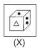Test: BITSAT Mock Test- 11 - Question 148

Select the correct mirror-image of the Figure (X) from amongst the given alternatives.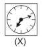Test: BITSAT Mock Test- 11 - Question 149

Each of the following questions consists of five Problem figures marked 1,2,3,4 and 5 followed by five Answer figures marked A,B,C,D and E . Select a figure from the Answer figures which will continue the same series as given in the Problem figures.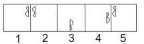Detailed Solution for Test: BITSAT Mock Test- 11 - Question 149

The symbol gets vertically inverted and laterally inverted alternately. It also moves in ACW direction through distances equal to two half-sides (of square boundary) and three half-sides alternately.

Test: BITSAT Mock Test- 11 - Question 150

Each of the following questions consists of five Problem figures marked 1,2,3,4 and 5 followed by five Answer figures marked A,B,C,D and E . Select a figure from the Answer figures which will continue the same series as given in the Problem figures.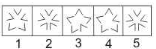Use Code STAYHOME200 and get INR 200 additional OFF Use Coupon Code